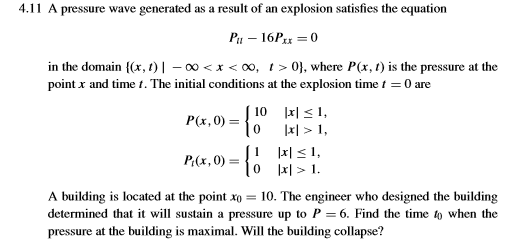# Question 4.11 A pressure wave generated as a result of an explosion satisfies the equation Pu - 16Pxx = 0 in the domain {(x,1) - 00<x< 0,1 >0}, where P(x, 1) is the pressure at the point x and time 1. The initial conditions at the explosion time 1 = 0 are 10 x < 1, P(x,0) = 1o x|>1, P(x,0) = {1 l sl. P:(x,0) = 10 x > 1. A building is located at the point Xo = 10. The engineer who designed the building determined that it will sustain a pressure up to P=6. Find the time to when the pressure at the building is maximal. Will the building collapse?